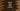# Python string zfill method explanation with example## Python string zfill() method:

The zfill method is an inbuilt method of Python string class. This method is used to add 0 to the left of a string to create another string of a specific length. This is a useful method if you want to pad zeroes to the left of a string.

In this post, we will learn how to use the zfill method of Python string with examples.

### Definition of zfill:

The zfill method is defined as like below:

``str.zfill(width)``

Here, width is the width of the final string. This value is required. The returned string will be of size width. It will pad 0’s to the beginning of the string if the size of the string is smaller than width.

### Return value of zfill:

The zfill() method returns a new string with 0’s padded at the start to make the length equal to width. If the length of the original string is equal to width or if it is greater than width, it will return the original string without any change.

### Example of zfill:

Let’s take an example of zfill:

``````s = 'hello'

print(s.zfill(0)) # hello
print(s.zfill(5)) # hello
print(s.zfill(8)) # 000hello``````

Here,

• For the first one, it returns the same string hello because the width value 0 is smaller than the string size i.e. 5
• For the second one, it again returns the same string hello because the width is equal to the string size i.e. 5
• The last one padded three ‘0’ before the string to make the size equal to 8.

### Example of zfill with positive and negative signs:

If we use zfill with positive or negative signs, it will put the sign to the left. This is useful if you want to use zfill with positive and negative numbers in string form.

``````str_arr = ['9981', '+9981', '+00', '+9981', '--98', '+++99']

for s in str_arr:
print(f'{s}.zfill(7): {s.zfill(7)}')``````

This example uses zfill with 7 as the width value on all elements of str_arr. it will print the below output:

``````9981.zfill(7): 0009981
+9981.zfill(7): +009981
+00.zfill(7): +000000
+9981.zfill(7): +009981
--98.zfill(7): -000-98
+++99.zfill(7): +00++99``````

If the string has + or - sign at the start of the string, it moves that to the start. If it has multiple plus or minus signs, it moves one to the left.

It works with only + and -, doesn’t work with others:

``````str_arr = ['*98', '&988', '_88', '\$888']

for s in str_arr:
print(f'{s}.zfill(7): {s.zfill(7)}')``````

It will print:

``````*98.zfill(7): 0000*98
&988.zfill(7): 000&988
_88.zfill(7): 0000_88
\$888.zfill(7): 000\$888``````

### ValueError:

zfill will throw a ValueError if we don’t provide the width.

``TypeError: str.zfill() takes exactly one argument (0 given)``

Let’s consider the below example:

``````s = ''

print(s.zfill())``````

It will raise TypeError:

``````Traceback (most recent call last):
File "example.py", line 3, in <module>
print(s.zfill())
TypeError: str.zfill() takes exactly one argument (0 given)``````